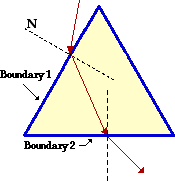Ray Optics: Refraction and Lenses

Refraction and Lenses: Audio Guided Solution

Problem 18:In a physics lab, Anna Litical is studying the path of red laser light through a equiangular glass prism (n = 1.52). She observes that the light enters one face of the glass prism (boundary 1), refracts and then exits a second face of the glass prism (boundary 2). In her usual inquisitive manner, she ponders the question "I wonder if I could find an angle of approach to boundary 1 which would cause the light to undergo total internal reflection at boundary 2?" Calculate the angle of incidence at boundary 1 which would cause this total internal reflection at boundary 2.

Audio Guided Solution

Click to show or hide the answer!

Habits of an Effective Problem Solver

• Read the problem carefully and develop a mental picture of the physical situation. If necessary, sketch a simple diagram of the physical situation to help you visualize it.
• Identify the known and unknown quantities and record them in an organized manner. Equate given values to the symbols used to represent the corresponding quantity - e.g., do = 24,8 cm; di = 16.7 cm; f = ???.
• Use physics formulas and conceptual reasoning to plot a strategy for solving for the unknown quantity.
• Identify the appropriate formula(s) to use.
• Perform substitutions and algebraic manipulations in order to solve for the unknown quantity.